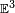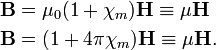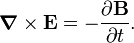# Magnetic induction

In physics, and more in particular in the theory of electromagnetism, magnetic induction (also known as magnetic flux density) describes a magnetic force (a vector) on a test object (for instance a small piece of iron) at every point in space. The magnetic induction is commonly denoted by B(r,t) and is a vector field, that is, it depends on position r and time t. In non-relativistic physics, the space on which B is defined is the three-dimensional Euclidean space$\mathbb{E}^3$—the infinite world that we live in. The field B is closely related to the magnetic field H. In fact, some authors refer to B as the magnetic field and to H as an auxiliary field.

The physical source of the field B can be

or combinations of these three. A magnetic field exists in the neighborhood of these sources. In general the strength of the magnetic field decreases as a low power of 1/R, the inverse of the distance R to the source.

A magnetic force can act on

The term magnetic flux density refers to the fact that B is magnetic flux per unit surface. This relationship is based on Faraday's law of magnetic induction.

The SI unit measuring the strength of B is T (tesla = weber/m2), and the Gaussian unit of B is G (gauss = maxwell/cm2) . One tesla is 10 000 gauss.

To give an indication of magnitudes: the magnetic field (or better: magnetic induction) of the Earth is about 0.5 G (50 μT). A horse shoe magnet is about 100 G. A medical MRI diagnostic machine typically supports a field of up to 2 T (20 kG). The strongest magnets in laboratories are currently about 30 T (300 kG).

## Note on nomenclature

Most textbooks on electricity and magnetism distinguish the magnetic field H and the magnetic induction B. Yet, in practice physicists and chemists almost always call B the magnetic field, which is because the term "induction" suggests an induced magnetic moment. Since an induced moment is usually not in evidence, the term induction is felt to be confusing. Among scientists phrases are common as: "This EPR spectrum was measured at a magnetic field of 3400 gauss", and "Our magnet can achieve magnetic fields as high as 20 tesla". That is, most scientists use the term "field" with units tesla or gauss, while strictly speaking, gauss and tesla are units of B. Some authors go one step further and reserve the name "magnetic field" for B and refer to H as the "auxiliary magnetic field".

## Relation between B and H

In vacuum (also known as the microscopic case, see Maxwell equations), in the absence of a magnetizable medium, the fields B and H are related as follows,\begin{align}\mathbf{B} &= \mu_0 \mathbf{H} \qquad \hbox{in SI units}\\\mathbf{B} &= \mathbf{H} \qquad\quad \hbox{in Gaussian units},\\\end{align}

where μ0 is the magnetic constant (equal to 4π⋅10−7 N/A2). Note that in Gaussian units the dimensions of H (Oer) and of B (G = gauss) are equal, 1 Oer = 1 G, although the units have an unrelated definition (Oer is based on the field of a solenoid, and G is magnetic flux/surface). In the absence of a magnetizable medium it is unnecessary to introduce both B and H, because they differ by an exact and constant factor (unity for Gaussian units and μ0 for SI units).

In the presence of a magnetizable medium the relation between B and H contains the magnetization M of the medium,\begin{align}\mathbf{B} = \mu_0 (\mathbf{H} + \mathbf{M})\qquad& \hbox{in SI units}\\ \mathbf{B} = \mathbf{H} + 4\pi \mathbf{M}\qquad\;\; &\hbox{in Gaussian units},\\\end{align}

In almost all non-ferromagnetic media, the magnetization M is linear in H,$\mathbf{M} = \boldsymbol{\chi} \mathbf{H} \quad \Longleftrightarrow \quad M_\alpha = \sum_{\beta = x,y,z} \chi_{\alpha\beta} H_\beta.$

For a magnetically isotropic medium the magnetic susceptibility tensor χ is a constant times the identity 3×3 matrix, χ = χm 1. For an isotropic medium the relation between B and H is in SI and Gaussian units, respectively,\begin{align}\mathbf{B} &= \mu_0(1 + \chi_m) \mathbf{H} \equiv \mu \mathbf{H} \\ \mathbf{B} &= (1 + 4\pi \chi_m) \mathbf{H} \equiv \mu\mathbf{H}.\\ \end{align}

The material constant μ, which expresses the "ease" of magnetization of the medium, is the magnetic permeability of the medium. In most non-ferromagnetic materials χm << 1 and consequently B ≈ μ0H (SI) or BH (Gaussian). For ferromagnetic materials the magnetic permeability μ can be sizeable (χm >> 1). In that case the magnetization of the medium greatly enhances the magnetic field.

The two macroscopic Maxwell equations that contain charges and currents, are equations for H and electric displacement D. This is a consequence of the fact that current densities J and electric fields E (due to charges) are modified by the magnetization M and the polarization P of the medium. In SI units the Maxwell equation for the magnetic field is:$\boldsymbol{\nabla} \times \mathbf{H} = \mathbf{J} + \frac{\partial \mathbf{D}}{\partial t}.$

The microscopic (no medium) form of this equation is obtained by eliminating D and H via D = ε0E and H = B0 (P = 0 and M = 0).

The two Maxwell equations that do not contain currents and charges give relations between the fundamental fields E and B, instead of between the auxiliary fields H and D. For instance, Faraday's induction law in SI units is,$\boldsymbol{\nabla} \times \mathbf{E} = - \frac{\partial \mathbf{B}}{\partial t} .$

This equation is valid microscopically (vacuum) as well as macroscopically (in presence of a medium).